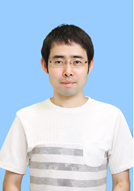## EnglishPage

 KONDO ShintaroTitle Associate Professor Department of Electrical, Electronic and Computer Engineering Applied Physics Course

#### Research fields

My research field is mathematical analysis of nonlinear partial differential equations. I have been studied Hasegawa-Wakatani equations which describe turbulence phenomena in nuclear fusion plasma. Recently I have been studied biological problems by using reaction-diffusion equations. I use mainly energy method and theory of Fourier series. For example, in plasma physics, many reduced model equations are proposed, and it is often difficult for mathematicians to judge which of those is better. Then mathematicians should research the standing points of plasma physicists. Although the standing points of plasma physicists and that of mathematicians are different, I want to find out the problems which are important for both plasma physicists and mathematicians, and research it.

#### Research Keywords

Partial Differential Equations,   Plasma,   Reaction-Diffusion Equations